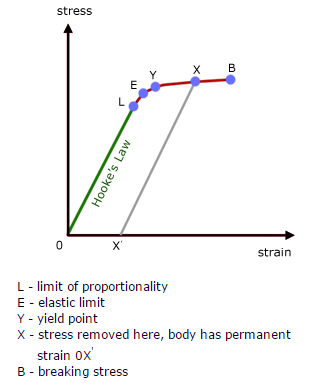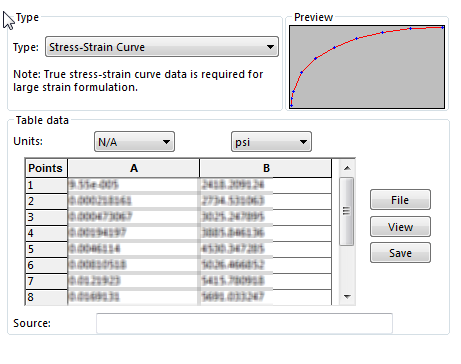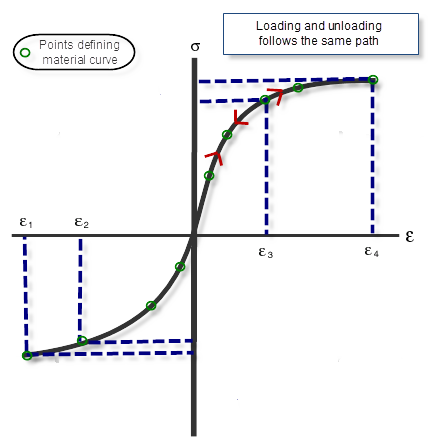In the first part of this blog series, we talked about the Linear Elastic material models which obey Hooke’s law to identify material behavior. To read part one, click here.

Hooke’s law is by far one of the most used principles of physics because, simply put, it works! Well, at least in most cases, but not all, because it is only an approximation of the actual material behavior and limited to the linear portion of the stress-strain graph.Since no material can be compressed beyond a certain minimum size, or stretched beyond a maximum size without permanent deformation, Hooke’s law is only valid up to the limit of proportionality (L). So basically, Hooke’s Law is an accurate approximation for most solid bodies, as long as the forces and deformations are small. In addition to that, as Hooke’s law is simply a linear approximation, the actual stress-strain graph of many materials do deviate from Hooke’s Law well before the elastic limit (E) point is reached. So the question is, when simulating your parts or assemblies, what if:

1. There are high loads or deformations (changes in stiffness)
2. You would like to see what happens to your parts after the limit of proportionality
3. You would like to see the exact behavior of your parts under load (no approximation)

To properly simulate analyses that fall under these “what if” scenarios, the Non Linear material model should be used.Note: The Nonlinear Elastic model will account for material behavior up to the yield point. In cases where the post yielding behavior (Y to X) is needed, the elasto-plastic model can be used. In the next blog for this series, we will talk about the 3 different Plasticity types which are Von Mises, Tresca, and Drucker Prager.

To be able to use the nonlinear elastic model, a stress-strain graph must be defined within SOLIDWORKS Simulation. This can be done by selecting the “Create stress-strain curve” on the material properties window. You can then input stress strain points to create the graph.For this material model to work, a continuous stress-strain curve should be plotted. This basically means the points should either be continuously increasing or decreasing in value. At times, data from physical material testing can provide points where the strain or stress point in value due to apparatus tolerance. These points should be omitted when being inputted into the program.A stress-strain curve can be defined in tension and compression, thus representing different material behavior to under tensile and compressive loads. If the compression portion of the curve is not specified, SOLIDWORKS Simulation will automatically assume that tension and compression would have to respond similarly.

Besides the stress strain curve, the material model will also need other properties such as Poisson’s ratio and mass density. The tensile strength is not required, but recommended. If the simulation has any thermal loads involved, a thermal expansion coefficient (CTE) can also be input.  The nonlinear material model also allows for the CTE, Poisson’s ratio and density to be temperature dependent, giving you more accurate results!